Reach Us+44-1522-440391
On the Representation Spaces of the Jordanian Plane | OMICS International
Journal of Generalized Lie Theory and Applications
All submissions of the EM system will be redirected to Online Manuscript Submission System. Authors are requested to submit articles directly to Online Manuscript Submission System of respective journal.

# On the Representation Spaces of the Jordanian Plane

Natalia K Iyudu*

Department of Pure Mathematics, Queen’s University Belfast, Belfast BT7 1NN, UK

Corresponding Author:
Natalia K Iyudu
Department of Pure Mathematics
Queen’s University Belfast, Belfast BT7 1NN, UK
E-mail: [email protected]

Received date: July 21, 2015; Accepted date: August 03, 2015; Published date: August 31, 2015

Citation: Iyudu NK (2015) On the Representation Spaces of the Jordanian Plane. J Generalized Lie Theory Appl S1:e001. doi:10.4172/generalized-theory-applications.S1-e001

Copyright: © 2015 Iyudu NK. This is an open-access article distributed under the terms of the Creative Commons Attribution License, which permits unrestricted use, distribution, and reproduction in any medium, provided the original author and source are credited.

Visit for more related articles at Journal of Generalized Lie Theory and Applications

We announce here a number of results concerning representation theory of the algebra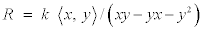, known as Jordanian plane (or Jordan algebra). We consider the question on 'classification' of finite-dimensional modules over the Jordan algebra. Complete description of irreducible components of the representation variety mod(R, n) is given for any dimension n. It is obtained on the basis of the stratification of this variety related to the Jordan normal form of Y. Any irreducible component of the representation variety contains only one stratum related to a certain partition of n and is the closure of this stratum. The number of irreducible components therefore is equal to the number of partitions of n.

As a preparation for the above result we describe the complete set of pairwise non-isomorphic irreducible modules Sa over the Jordan algebra, and the rule how they could be glued to indecomposables. Namely, we show that Ext1(Sa, Sb) = 0, if a ≠ b.

We study then properties of the image algebras in the endomorphism ring. Particularly, images of representations from the most important stratum, corresponding to the full Jordan block Y. This stratum turns out to be the only building block for the analogue of the Krull-Remark-Schmidt decomposition theorem on the level of irreducible components. Along this line we establish an analogue of the Gerstenhaber–Taussky–Motzkin theorem on the dimension of algebras generated by two commuting matrices. Another fact concerns with the tame-wild question for those image algebras. We show that all image algebras of n-dimensional repre-sentations are tame for n ≤ 4 and wild for n ≥ 5.

We consider here the quadratic algebra given by the presentation. This algebra appears in various different contexts in mathematics and physics. First of all, it is a kind of quantum plane: one of the two Auslander regular algebras of global dimension two, as it is mentioned in the Artin and Shelter paper . The other one is the usual quantum plane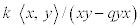. It served as one of basic examples for the foundation of noncommutative geometry . There were also studies of deformations of GL(2) analogous to GLq(2) with respect to R in 80-90th in Manin’s ’Quantum group’ [3,4], where this algebra appeared under the name Jordan algebra.

This algebra is also a simplest element in the class of RIT (relativistic internal time) algebras. The latter was defined and investigated [5-9]. The class of RIT algebras arises from a modification of the Poincare algebra of the Lorenz group SO(3,1) by means of introducing the additional generator corresponding to the relativistic internal time. The algebra R above is a RIT algebra of type (1,1). Our studies of this algebra are partially reported “Classification of finite dimensional representations of one noncommutative quadratic algebra” .

Let us mention that R is a subalgebra of the first Weyl algebra A1. The latter has no finite dimensional representations, but R turns out to have quite a rich structure of them. Category of finite dimensional modules over R contains, for example, as a full subcategory mod GP (n, 2), where GP (n, 2) is the Gelfand–Ponomarev algebra  with the nilpotency degrees of variables x and y, n and 2 respectively. On the other hand we show that R is residually finite dimensional.

We are interested here in representations over an algebraically closed field k of characteristic 0. Sometimes we just suppose k =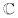, this will be pointed out separately. We denote throughout the category of all R-modules by Mod R, the category of finite dimensional R-modules by mod R and ρn stands for an n-dimensional representation of R.

The most important question on finite dimensional representations one can ask, is to classify them. This question may be solved directly by parametrization of isoclasses of indecomposable modules, for example, for tame algebras. But when the algebra is wild the problem turns to a description of orbits under GLn action by simultaneous conjugation in the space mod(R,n) of n-dimensional representations. One can think of the latter space also as of a variety of tuples of n × n matrices (corresponding to generators) satisfying the defining relations of the algebra R.

It is commonly understood that the first step in the study of this variety should be the description of its irreducible components. This approach leads to such famous results of this kind as Kashiwara-Saito  description of the irreducible components of Lusztig’s nilpotent variety via the crystal basis .

We show that irreducible components of the representation space mod(R, n) of the Jordan algebra could be completely described for any dimension n.

The first key point for this description is the choice of a stratification of the Jordan variety. We choose a stratum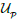corresponding to the partition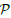= (n1,…, nk), n = n1 +…+ nk, n1 ≥ … ≥ nk, consisting of the pairs of matrices (X, Y ), where Y has the Jordan normal form defined byand X, Y satisfy the defining relation.

Theorem 12.1 Any irreducible component Kj of the representation variety mod(R,n) of the Jordan algebra contains only one stratumfrom the stratification related to the Jordan normal form of Y , and is the closure of this stratum.

The number of irreducible components of the variety mod(R, n) is equal to the number of the partitions of n.

The variety mod (R, n) is equidimensional, the dimension of components is n2.

The importance of examples of algebras for which the irreducible components of mod (R, n) could be described for each n was emphasized  and it is mentioned there that known cases are restricted to algebras of finite representation type (i.e., there are only finitely many isomorphism classes of indecomposable R modules) and one example of infinite representation type . There is some similarity between the algebra generated by the pair of nilpotent matrices annihilating each other considered  and the Jordan algebra, but while in the case of  variables x and y act ’independently’, there is much more interaction in the case of Jordan algebra, which makes the analysis in a sense more difficult.

We also can answer the question, in which irreducible components, module in general position is indecomposable.

Corollary: Only the irreducible component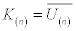which is the closure of the stratum corresponding to the trivial partition of n (the full block Y) contains an open dense subset consisting of indecomposable modules.

First, we derive some properties of algebras which are images for representations of R in the endomorphism ring. We show that they are basic algebras, that is their semisimple parts are direct sums of r copies of the field, where r is the number of different eigenvalues of X = ρn(x). This allows associating to any representation a quiver of its image algebra, in a conventional way. This leads to a rough classification of reps by these quivers. It turns out that indecomposable modules have either a typical wild quiver with one vertex and two loops or the quiver with one vertex and one loop. A simple, but important fact is that Y = ρn(y) is nilpotent for any ρnmod R. Note that this is not necessarily the case when the characteristic of the basic field is not zero. We describe the group of automorphisms of the Jordan algebra, in order to introduce later the notion of auto-equivalence of modules: equivalence up to automorphisms of the algebra R. We describe a complete set of prime ideals of R and point out which of them are primitive.

We show that all irreducible modules are one dimensional: Sα = (α, 0), and describe all finite dimensional modules, subject to the Jordan normal form of Y. We study indecomposable modules and derive the rule how one could glue irreducibles together: Ext1(Sα, Sβ) = 0, if α ≠ β. These provide us with enough information to suggest a stratification of the variety mod (R, n) related to the Jordan normal form of Y and to prove results on irreducible components.

Results on the structure of representation variety for R show an exceptional role of the strata related to the full Jordan block Y, since they turn out to be the only building blocks in the analogue of the Krull- Remark-Schmidt decomposition theorem on the level of irreducible components. Another evidence of the special role of this stratum is the following. We prove an analogue of the Gerstenhaber-Taussky- Motzkin theorem [16,17] on the dimension of algebras generated by two commuting matrices. We show that the dimension of image algebras of representations of R does not exceed n(n + 2)/4 for even n and (n + 1)2/4 for odd n and this estimate is attained in the stratum related the full Jordan block Y.

Finally, we fulfill a more detailed study of tame-wild questions for image algebras in the strata related to the full Jordan block Y. From the above results we see that any algebra which is an image of indecomposable representation ρn is a local complete algebra. Hence after developing some technique, which allows writing down defining relations in the image-algebra, we apply Ringel’s classification  of local complete algebras and get that all image-algebras are tame for n ≤ 4 and for n ≥ 5 they are wild .

#### Acknowledgements

The work on this circle of questions was started in 2003-2004 during my visit at the Max-Planck-Institute for Mathematics in Bonn. Most of results apart from those on the irreducible components appeared in the MPI preprint. I am thankful to this institution for the support and hospitality and to many colleagues with whom I have been discussing ideas related to this work.

#### References

Select your language of interest to view the total content in your interested language

### Article Usage

• Total views: 12048
• [From(publication date):
specialissue-2015 - Aug 23, 2019]
• Breakdown by view type
• HTML page views : 8114
• PDF downloads : 3934

## Post your commentCan't read the image? click here to refresh
###### Peer Reviewed Journals

Make the best use of Scientific Research and information from our 700 + peer reviewed, Open Access Journals

International Conferences 2019-20

Meet Inspiring Speakers and Experts at our 3000+ Global Annual Meetings

Top